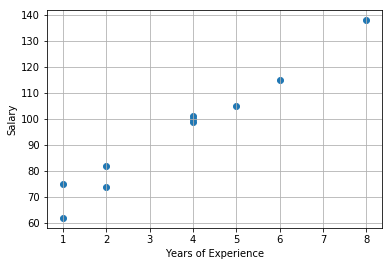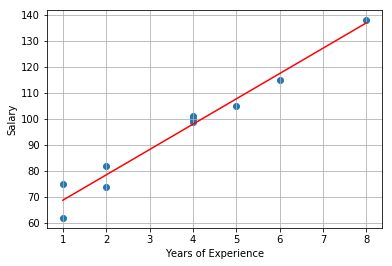# Linear Regression / CSC 578D / Fall 2018¶

### Notes:¶

1. See notes by hand here.

Author: Andreas P. Koenzen akoenzen@uvic.ca
Version: 0.1

In :
import pandas as pd
import numpy as np
import matplotlib.pyplot as plt

from IPython.core.interactiveshell import InteractiveShell
InteractiveShell.ast_node_interactivity = "all"


### Salary-Experience example:¶

• E = Experience in years. ($X$ = Predictor or Feature or Independent Variable)
• S = Salary in thousands of CAD. ($Y$ = Response or Outcome or Dependent Variable) Not to be confused with the Predicted Value $\hat{Y}$.

The data for this notebook is artificially generated by sampling from a Uniform distribution. Use the Gaussian distribution to sample values for C to compute the value of Y in order to introduce more variation.

In :
# generate the data
rng = np.random.RandomState(1) # seed the random function to obtain the same numbers
sample_number = 10

# load data into X and Y
X = 10 * (rng.rand(sample_number) + 0.1)
Y = 10 * (1.0 * X + 5.0 + rng.rand(sample_number))

# convert the data to integers
X = X.astype(int)
Y = Y.astype(int)

# plot the data
plt.scatter(X, Y)
plt.grid(True)
plt.xlabel('Years of Experience')
plt.ylabel('Salary')

Out:
<matplotlib.collections.PathCollection at 0x119587748>
Out:
Text(0.5,0,'Years of Experience')
Out:
Text(0,0.5,'Salary')In :
print('Values:')
data = pd.DataFrame({'X': X, 'Y': Y})
data

Values:

Out:
X Y
0 5 105
1 8 138
2 1 62
3 4 99
4 2 74
5 1 75
6 2 82
7 4 100
8 4 101
9 6 115

### Compute the weights of the line:¶

Do N iterations and compute the error.

In :
debug = False
iterations = 500
kappa = 0.1

# initialize vector w to all 1.0
w = np.array([[1.0, 1.0]])
# w
E = 0

for k in range(0, iterations):
# build the x vector. remember that it is the dummy value and the value of feature N.
# in this case it should be a 2D vector.
x0 = np.ones((len(X), 1), dtype=float)
x = np.hstack([x0, X[:, np.newaxis]])
if k + 1 == iterations and debug:
x

y = Y[:, np.newaxis]

# compute the Mean Square Error
E = (1 / (2 * len(X))) * np.sum(((y - (x @ w.T)) ** 2), axis=0, keepdims=True)
E = np.asscalar(E)
if k + 1 == iterations and debug:
E
if (k + 1) % 50 == 0:
print("Iteration: {0} => Error: {1}".format(k + 1, E))

# re-compute the weights using gradient descent
g = (1 / len(X)) * np.sum(((y - (x @ w.T)) * x), axis=0, keepdims=True)
if k + 1 == iterations and debug:
g

# update the weights
w = w + (kappa * g)

w
E

Iteration: 50 => Error: 40.86086176233402
Iteration: 100 => Error: 10.17609043828013
Iteration: 150 => Error: 7.545350515968571
Iteration: 200 => Error: 7.318029874926935
Iteration: 250 => Error: 7.298387145734406
Iteration: 300 => Error: 7.296689821596124
Iteration: 350 => Error: 7.296543156170857
Iteration: 400 => Error: 7.296530482842009
Iteration: 450 => Error: 7.296529387742303
Iteration: 500 => Error: 7.296529293114964

Out:
array([[59.0387855 ,  9.74625719]])
Out:
7.296529293114964

### Plot the line:¶

In :
# plot the data
plt.scatter(X, Y)
plt.grid(True)
plt.xlabel('Years of Experience')
plt.ylabel('Salary')

# plot line equation
plt.plot(
range(1, 9),
[(k * np.squeeze(w) + np.squeeze(w)) for k in range(1, 9)],
c= "red"
)

Out:
<matplotlib.collections.PathCollection at 0x1193be5c0>
Out:
Text(0.5,0,'Years of Experience')
Out:
Text(0,0.5,'Salary')
Out:
[<matplotlib.lines.Line2D at 0x1191851d0>]